Examples from NCERT Book

Class 9
Chapter 10 Class 9 - Gravitation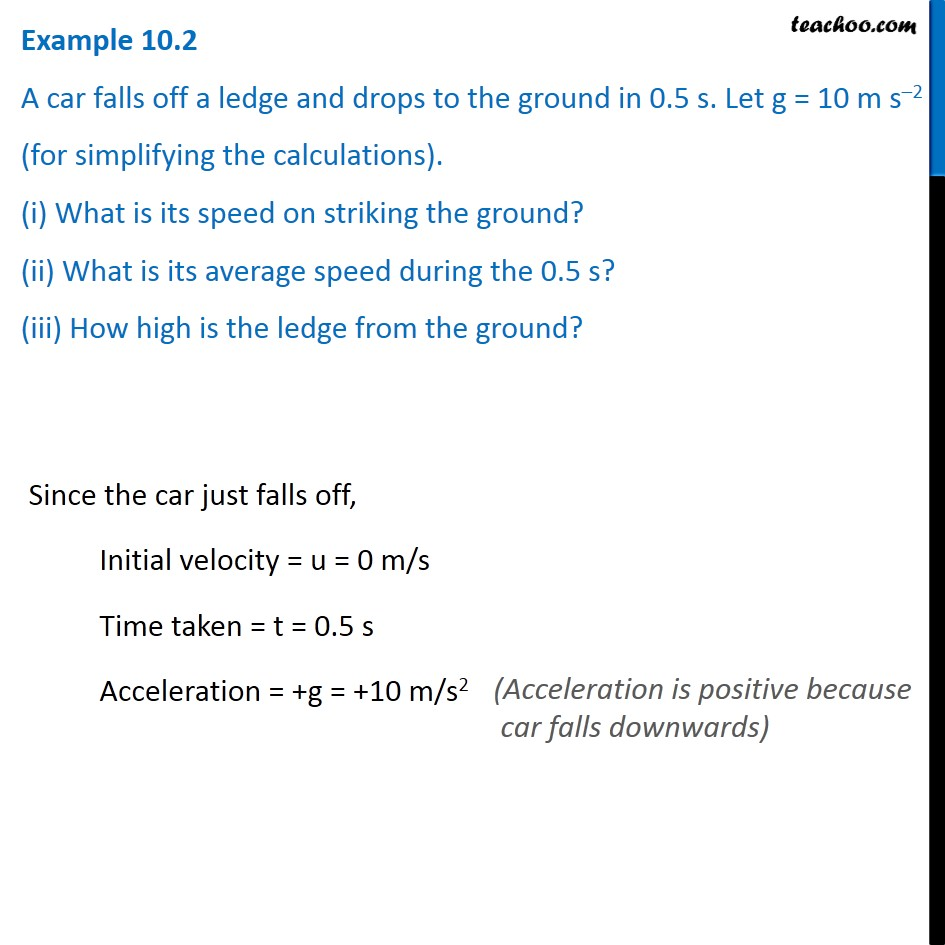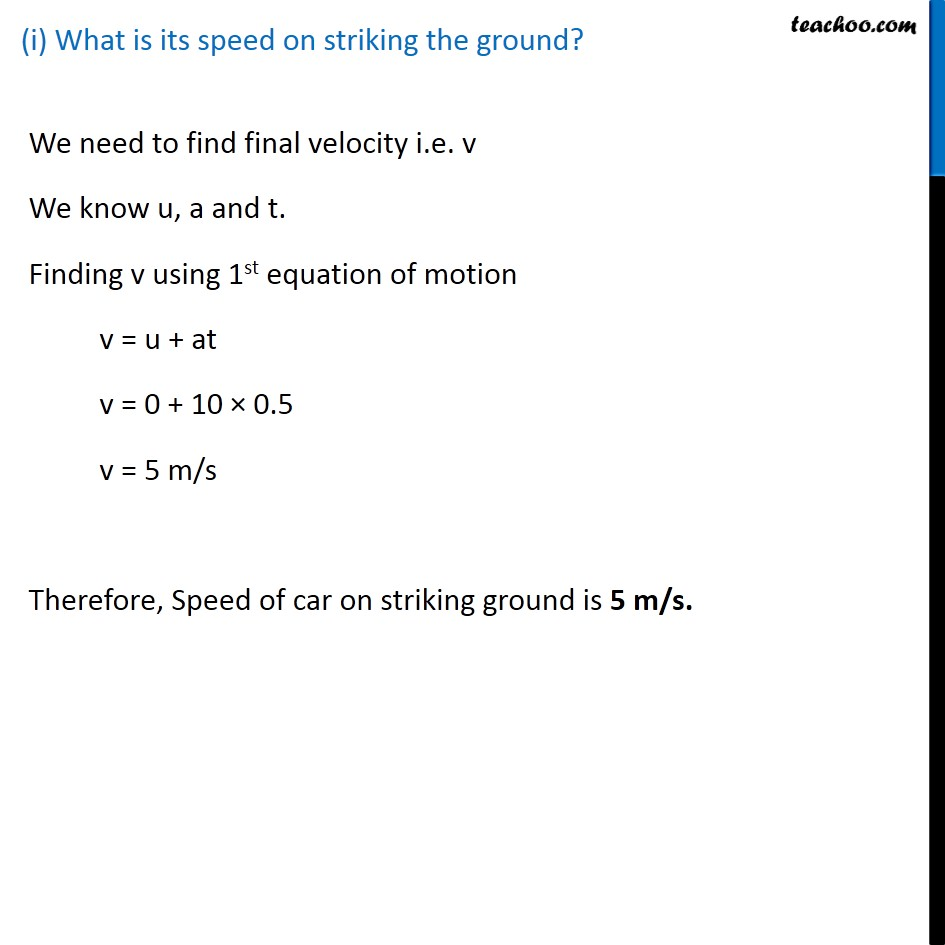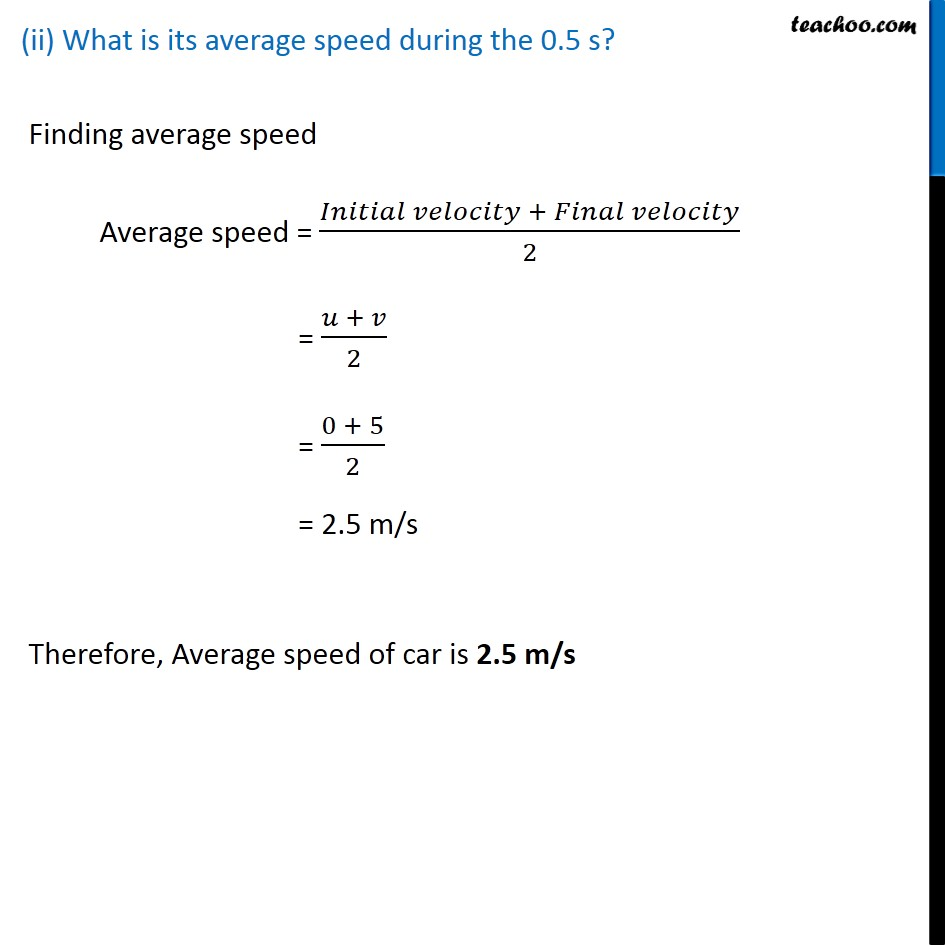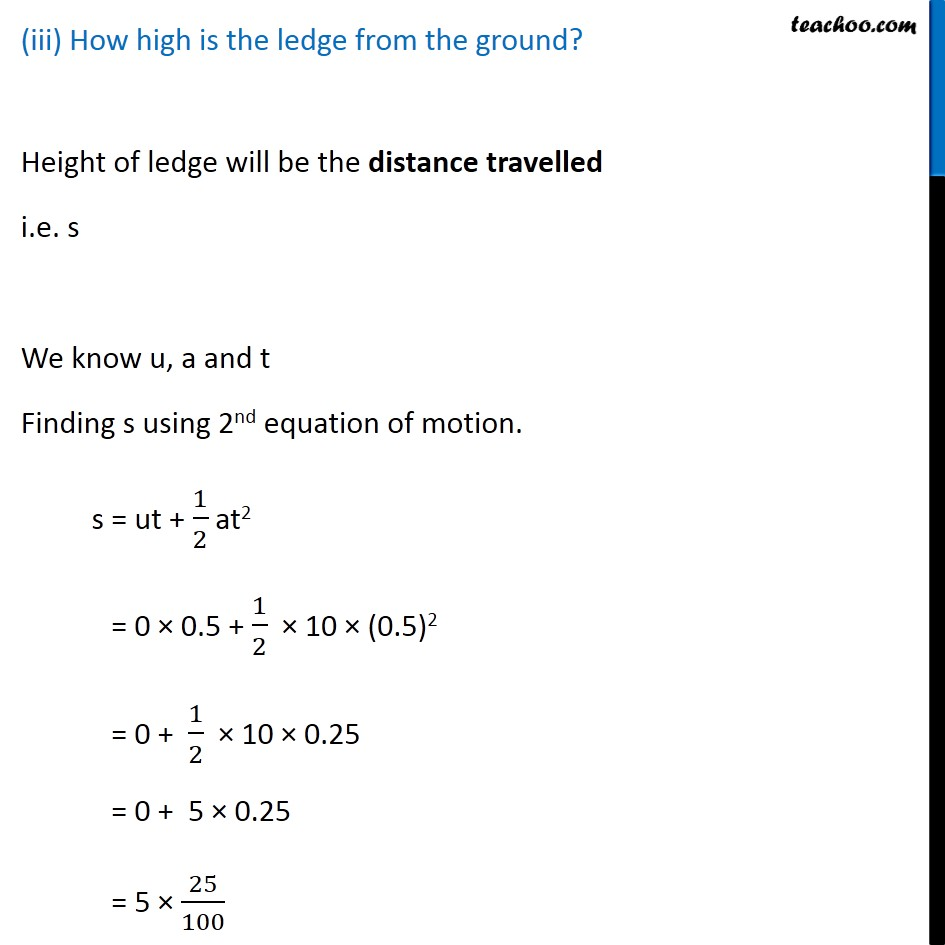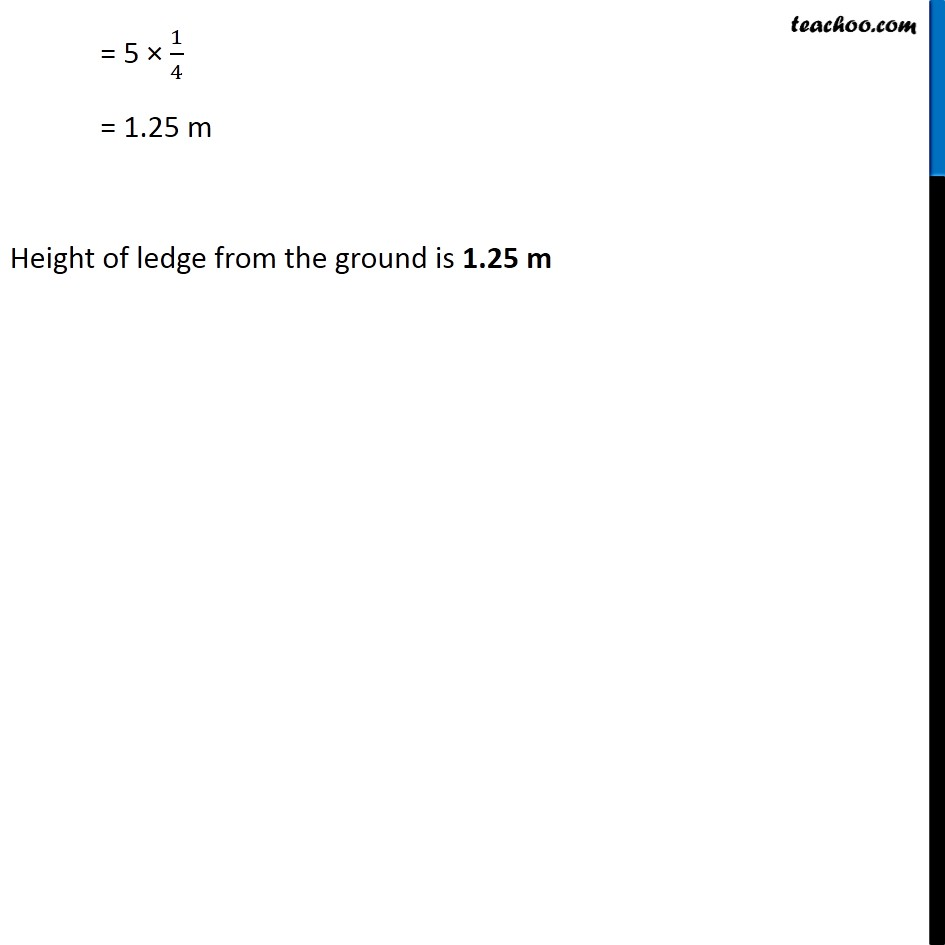Learn in your speed, with individual attention - Teachoo Maths 1-on-1 Class

### Transcript

Example 10.2 A car falls off a ledge and drops to the ground in 0.5 s. Let g = 10 m s–2 (for simplifying the calculations). (i) What is its speed on striking the ground? (ii) What is its average speed during the 0.5 s? (iii) How high is the ledge from the ground? Since the car just falls off, Initial velocity = u = 0 m/s Time taken = t = 0.5 s Acceleration = +g = +10 m/s2 (Acceleration is positive because car falls downwards) (i) What is its speed on striking the ground? We need to find final velocity i.e. v We know u, a and t. Finding v using 1st equation of motion v = u + at v = 0 + 10 × 0.5 v = 5 m/s Therefore, Speed of car on striking ground is 5 m/s. (ii) What is its average speed during the 0.5 s? Finding average speed Average speed = (𝐼𝑛𝑖𝑡𝑖𝑎𝑙 𝑣𝑒𝑙𝑜𝑐𝑖𝑡𝑦 + 𝐹𝑖𝑛𝑎𝑙 𝑣𝑒𝑙𝑜𝑐𝑖𝑡𝑦)/2 = (𝑢 + 𝑣)/2 = (0 + 5)/2 = 2.5 m/s Therefore, Average speed of car is 2.5 m/s (iii) How high is the ledge from the ground? Height of ledge will be the distance travelled i.e. s We know u, a and t Finding s using 2nd equation of motion. s = ut + 1/2 at2 = 0 × 0.5 + 1/2 × 10 × (0.5)2 = 0 + 1/2 × 10 × 0.25 = 0 + 5 × 0.25 = 5 × 25/100 = 5 × 1/4 = 1.25 m Height of ledge from the ground is 1.25 m